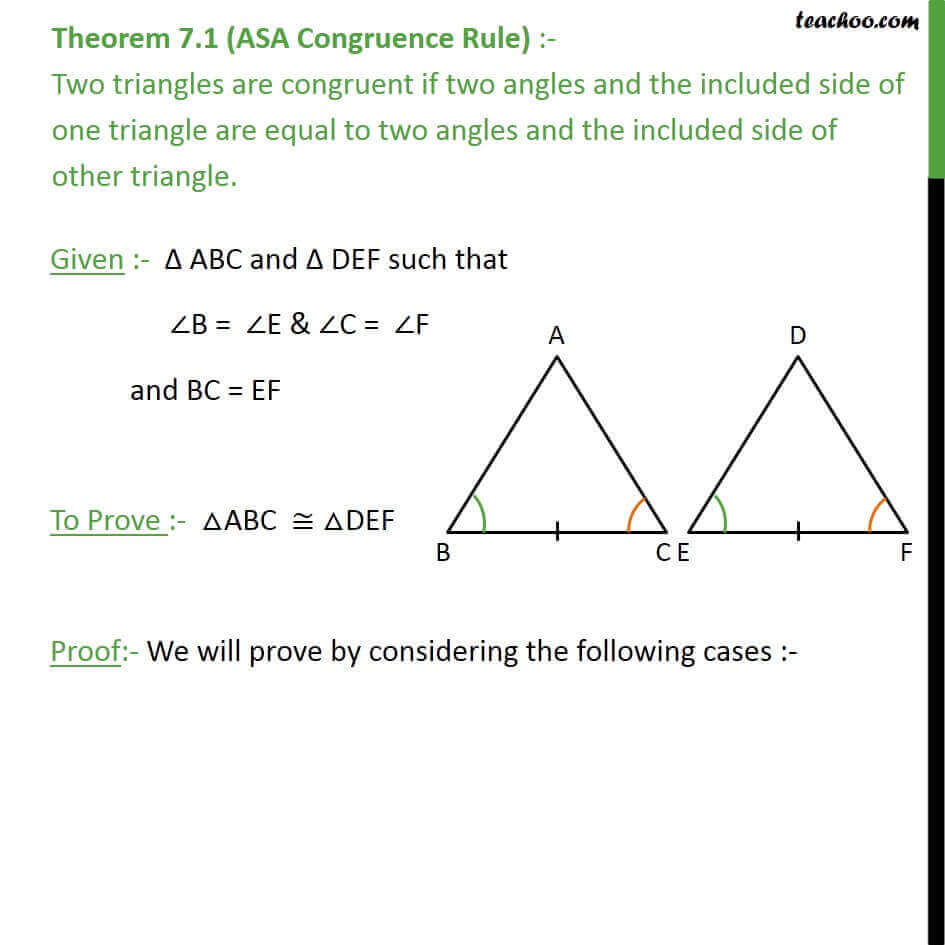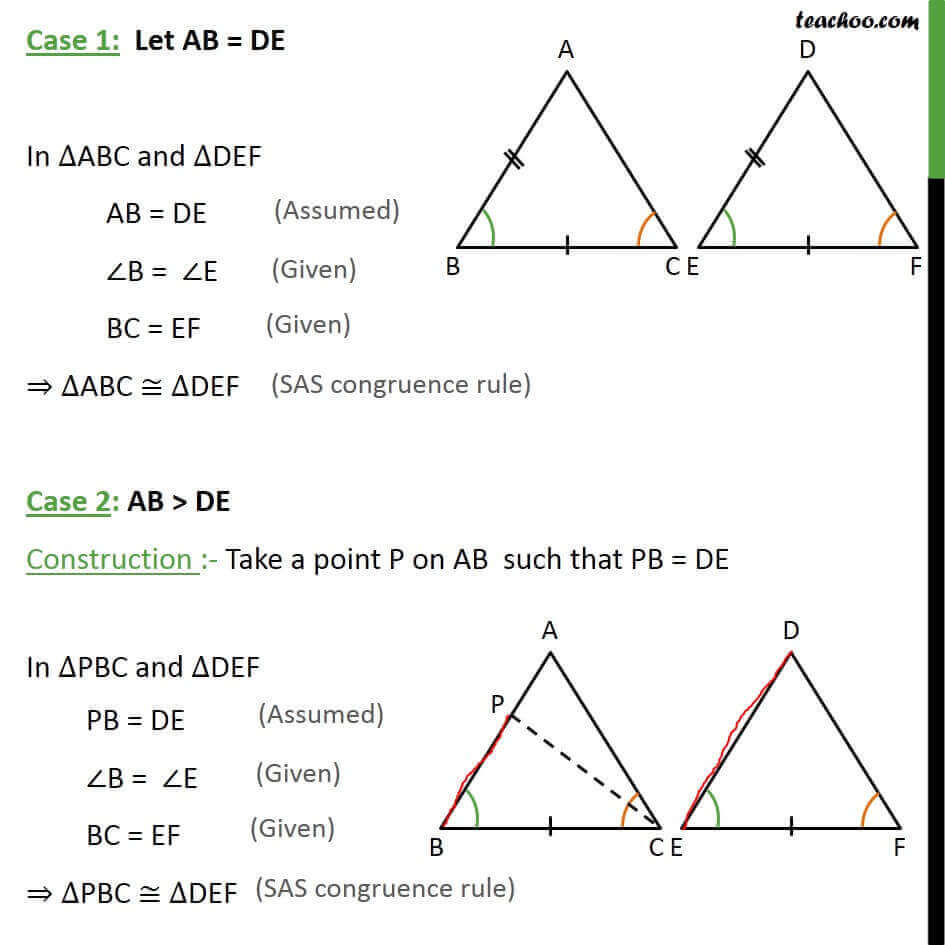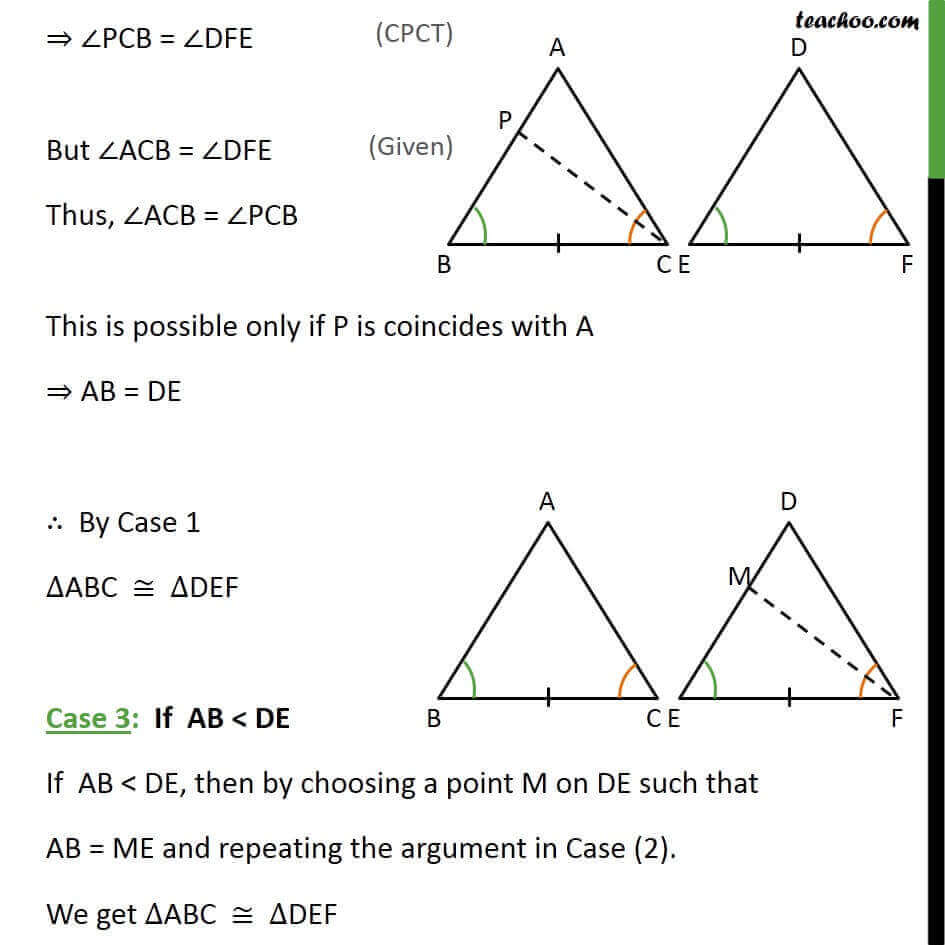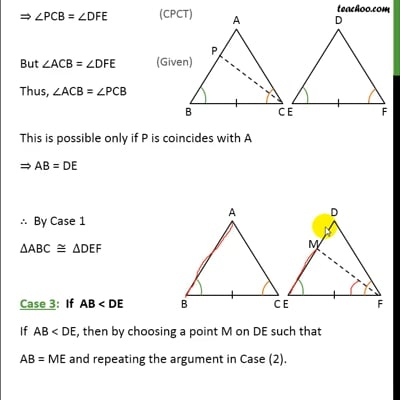Theorems

Chapter 7 Class 9 Triangles
Serial order wiseThis video is only available for Teachoo black users

Learn in your speed, with individual attention - Teachoo Maths 1-on-1 Class

### Transcript

Theorem 7.1 (ASA Congruence Rule) :- Two triangles are congruent if two angles and the included side of one triangle are equal to two angles and the included side of other triangle. Given :- ABC and DEF such that B = E & C = F and BC = EF To Prove :- ABC DEF Proof:- We will prove by considering the following cases :- Case 1: Let AB = DE In ABC and DEF AB = DE B = E BC = EF ABC DEF Case 2: AB > DE Construction :- Take a point P on AB such that PB = DE In PBC and DEF PB = DE B = E BC = EF PBC DEF PCB = DFE But ACB = DFE Thus, ACB = PCB This is possible only if P is coincides with A AB = DE By Case 1 ABC DEF Case 3: If AB < DE If AB < DE, then by choosing a point M on DE such that AB = ME and repeating the argument in Case (2). We get ABC DEF